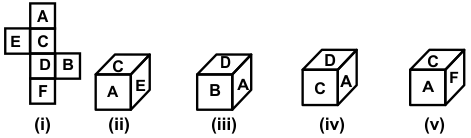# CREST Reasoning Olympiad Class 6 Sample Papers

REGISTER NOW

## Syllabus:

Section 1: Series Completion and Inserting the Missing Character, Analogy and Classification, Blood Relations, Coding - Decoding, Direction Sense Test, Logical Venn Diagram, Alpha Numeric Sequence Puzzle, Number, Ranking and Time Sequence Test, Logical Sequence of Words, Alphabet Test, Mathematical Operations, Analytical Reasoning, Mirror Images and Water Images, Embedded Figures, Figure Formation, Construction of Squares, Grouping of Identical Figures, Figure Matrix, Paper Folding and Paper Cutting, Cubes and Dice, Dot Situations.

Achievers Section: Higher Order Thinking Questions - Syllabus as per Section 1

 Q.1 A solid cube is divided into 27 smaller cubes of equal volume. If two opposite faces of this bigger cube are painted with different colours and remaining faces are left unpainted, then how many smaller cubes are obtained which have no faces painted?
 Q.2 If A means '+', B means '-' C means '×' and D means '÷' then 18 C 14 A 6 B 16 D 4 =?
 Q.3 Choose the box that will be formed by folding the sheet of paper given in figure(i).Q.4 Here two words are given which are related to each other in a particular manner and you have to find the word from the given alternatives which bears the exact same relationship to the third word, as the first two bears. 'Flower' is related to 'Petal' in the same way 'Book' is related to ______.
 Q.5 How many such letters are there in the word 'CATEGORY' each of which is as far away from the beginning of the words when they are arranged in alphabetical order?
 Q.6 There is a certain relationship between the pair of words given on either side of (::); identify the relationship of the given pair and find the matching term: EGIK : LJHF :: SUWY : ?
 Q.7 In the following question, one number is wrong in the series. Find out the number. 4, 5, 7, 10, 14, 18, 25, 32
 Q.8 Select the related word from the given alternatives: Milk : Booth :: Petrol : ?
 Q.9 In a certain code language, “PILLOW” is written as “WOLLIP”, “CHAIR” is written as “RIAHC”. Then “TABLE” will be written as:
 Q.10 B is the father of Q, B has only two children. Q is the brother of R. R is the daughter of P. A is the granddaughter of P and S is the father of A. How is S related to Q?

Sample PDF of CREST Reasoning Olympiad for Class 6: